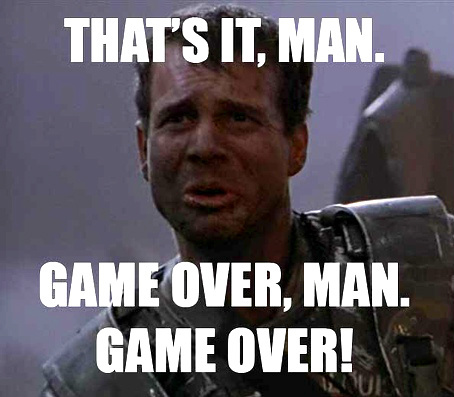# 🎲 Would You LIKE to Play a Game? 🎮

Grazie!

@wisconsinplatt = 1980 points

@jsroberts = 800 points

@Ignatius = 20 points

@Markdow = 10 points

@daneel = 30 points

@Melizmatic = 20 points

@dave_b = 880 points

@OtherMichael = 40 points

1 Like

20 just to even things out to a nice round 2000.

1 Like

Sheesh man, you’re doing a Bill Paxton all over this thing:@null = 45 points

@wisconsinplatt = 2000 points

@jsroberts = 800 points

@Ignatius = 20 points

@Markdow = 10 points

@daneel = 30 points

@Melizmatic = 20 points

@dave_b = 880 points

@OtherMichael = 50 points

1 Like

@Melizmatic - I’ve only posted 4 10-pointers, so…

1 Like

I must have double counted; thanks for your honesty.

1 Like

How ’ bout I take off and nuke it from orbit?

10

25

255 … but lunch hour is over

2 Likes

You have a bookmarklet or scraper, don’t you.

2 Likes

1 Like@null = 45 points

@wisconsinplatt = 2255 points

@jsroberts = 800 points

@Ignatius = 20 points

@Markdow = 10 points

@daneel = 30 points

@Melizmatic = 20 points

@dave_b = 880 points

@OtherMichael = 50 points, now

1 Like

25s

10s

155

That was a long conference call

3 Likes

When tallying WP’s points:@null = 45 points

@wisconsinplatt = 2410 points

@jsroberts = 800 points

@Ignatius = 20 points

@Markdow = 10 points

@daneel = 30 points

@Melizmatic = 20 points

@dave_b = 880 points

@OtherMichael = 50 points

2 Likes

Some call it exercise

1 Like

Try this next time

3 Likes

75

1 Like

Talking about Fight Club in a public forum, eh? Hmmm.

3 Likes
3 Likes

10s

25s

385

2 Likes

@null = 45 points

@wisconsinplatt = 2795 points

@jsroberts = 800 points

@Ignatius = 20 points

@Markdow = 10 points

@daneel = 30 points

@Melizmatic = 20 points

@dave_b = 880 points

@OtherMichael = 50 points

Soooooo… what kind of avatar would you like, Mr. Over~Achiever?

I might as well ask now…

3 Likes